Ex 6.2

Chapter 6 Class 12 Application of Derivatives
Serial order wise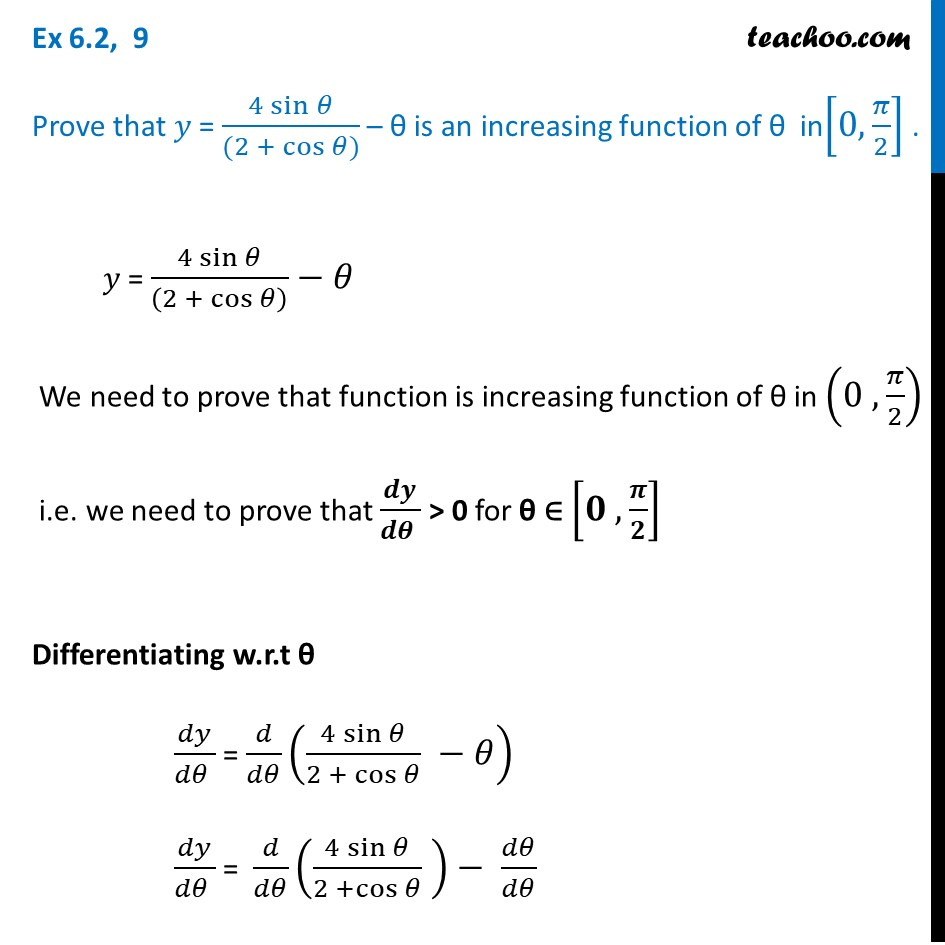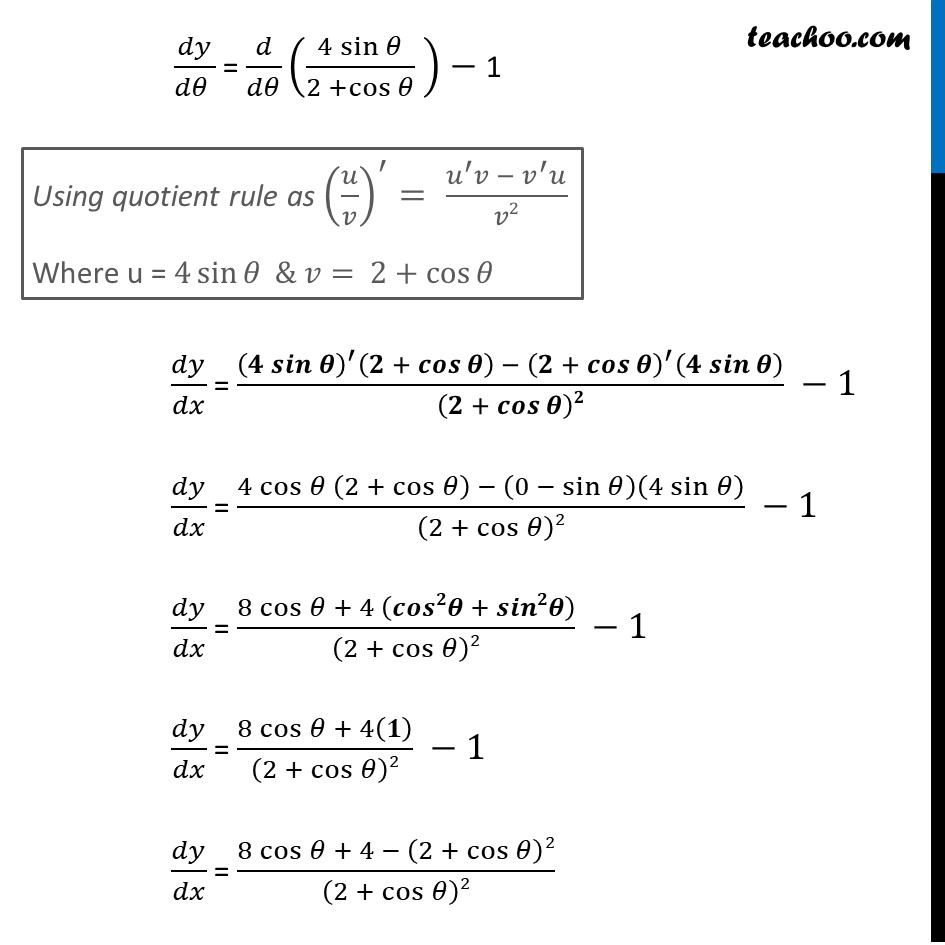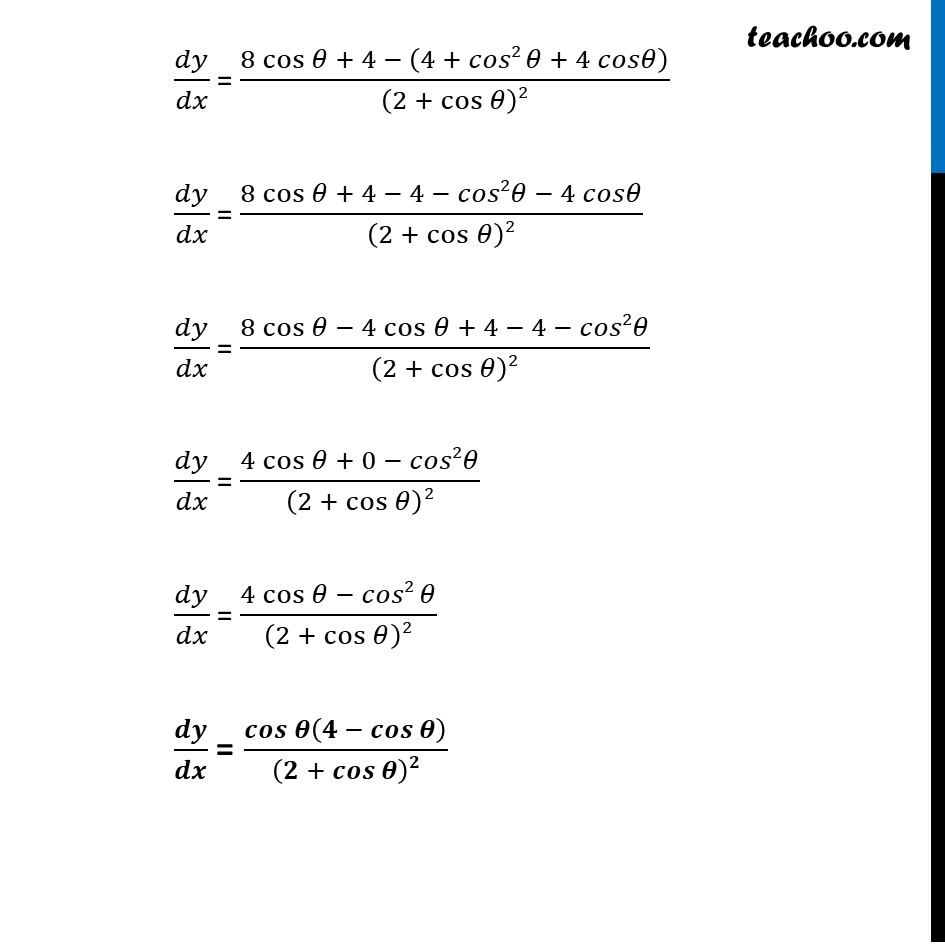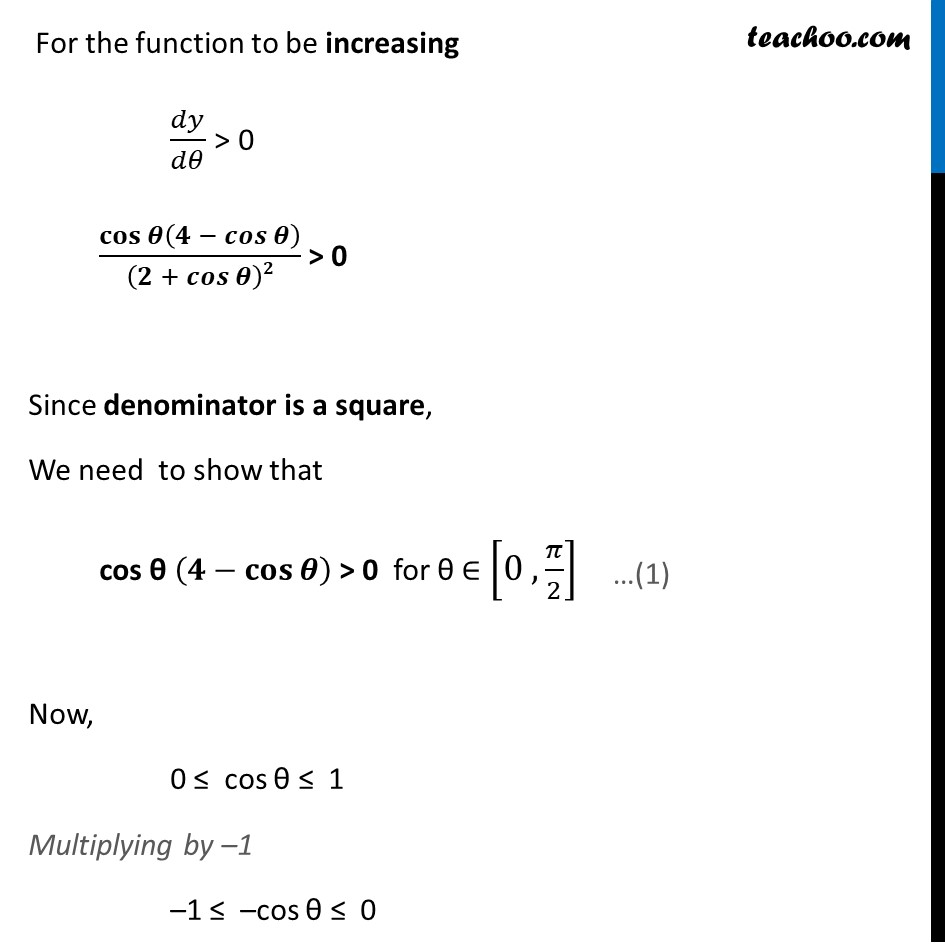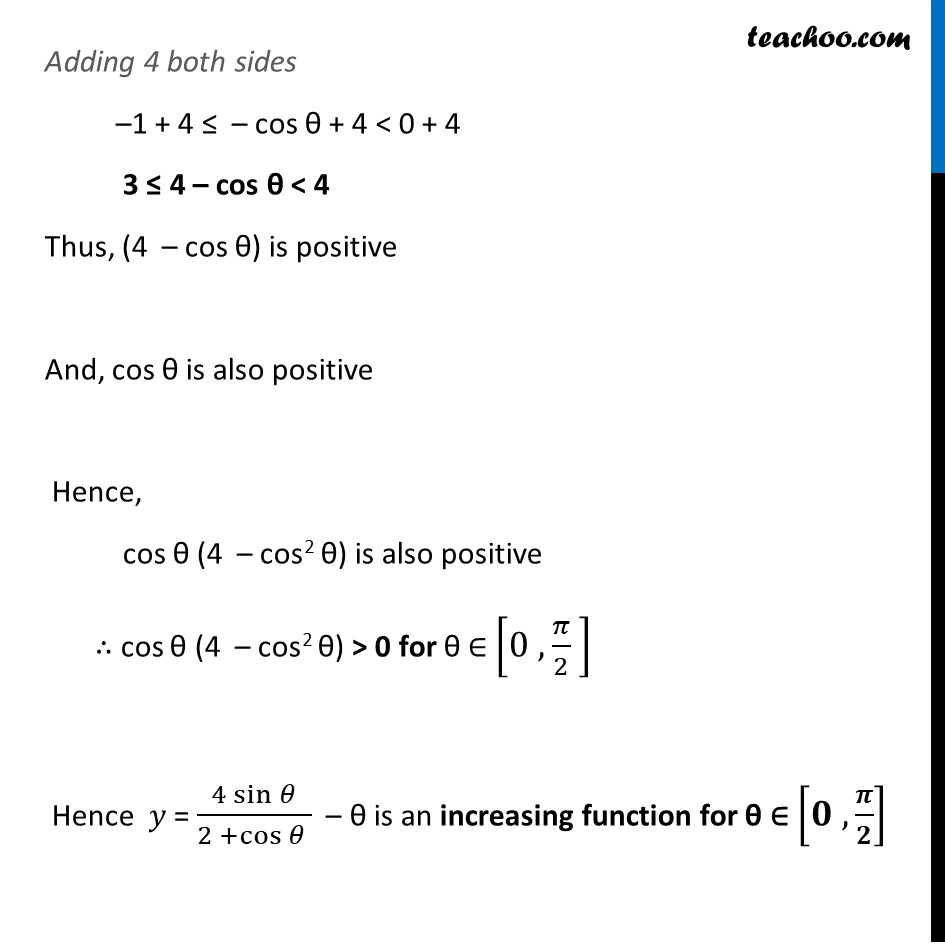Learn in your speed, with individual attention - Teachoo Maths 1-on-1 Class

### Transcript

Ex 6.2, 9 Prove that 𝑦 = (4 sin⁡𝜃)/((2 +〖 cos〗⁡〖𝜃)〗 ) – θ is an increasing function of θ in[0,𝜋/2] . 𝑦 = (4 sin⁡𝜃)/((2 + cos⁡𝜃 ) )−𝜃 We need to prove that function is increasing function of θ in (0 , 𝜋/2) i.e. we need to prove that 𝒅𝒚/(𝒅𝜽 ) > 0 for θ ∈ [𝟎 , 𝝅/𝟐] Differentiating w.r.t θ 𝑑𝑦/(𝑑𝜃 ) = 𝑑/𝑑𝜃 ((4 sin⁡𝜃)/(2 + cos⁡𝜃 ) −𝜃) 𝑑𝑦/(𝑑𝜃 ) = 𝑑/𝑑𝜃 ((4 sin⁡𝜃)/(2 +cos⁡𝜃 ) )− 𝑑𝜃/𝑑𝜃 𝑑𝑦/(𝑑𝜃 ) = 𝑑/𝑑𝜃 ((4 sin⁡𝜃)/(2 +cos⁡𝜃 ) )− 1 𝑑𝑦/𝑑𝑥 = ((𝟒 𝒔𝒊𝒏⁡𝜽 )^′ (𝟐 + 𝒄𝒐𝒔⁡𝜽 ) − (𝟐 + 𝒄𝒐𝒔⁡𝜽 )^′ (𝟒 𝒔𝒊𝒏⁡𝜽 ))/(𝟐 + 𝒄𝒐𝒔⁡𝜽 )𝟐 −1 𝑑𝑦/𝑑𝑥 = (4 cos⁡𝜃 (2 + cos⁡𝜃 ) − (0 − sin⁡𝜃 )(4 sin⁡𝜃 ))/(2 + cos⁡𝜃 )2 −1 𝑑𝑦/𝑑𝑥 = (8 cos⁡〖𝜃 + 4 (𝒄𝒐𝒔𝟐𝜽 + 𝒔𝒊𝒏𝟐𝜽)〗)/(2 + cos⁡𝜃 )2 −1 𝑑𝑦/𝑑𝑥 = (8 cos⁡〖𝜃 + 4(𝟏)〗)/(2 + cos⁡𝜃 )2 −1 𝑑𝑦/𝑑𝑥 = (8 cos⁡〖𝜃 + 4〗 − (2 + cos⁡𝜃 )2)/(2 + cos⁡𝜃 )2 Using quotient rule as (𝑢/𝑣)^′= (𝑢^′ 𝑣 − 𝑣^′ 𝑢)/𝑣2 Where u = 4 sin⁡𝜃 & 𝑣= 2+cos⁡𝜃 𝑑𝑦/𝑑𝑥 = (8 cos⁡〖𝜃 + 4〗 − (4 + 𝑐𝑜𝑠2 𝜃 + 4 𝑐𝑜𝑠𝜃))/(2 + cos⁡𝜃 )2 𝑑𝑦/𝑑𝑥 = (8 cos⁡〖𝜃 + 4〗 − 4 − 𝑐𝑜𝑠2𝜃 − 4 𝑐𝑜𝑠𝜃)/(2 + cos⁡𝜃 )2 𝑑𝑦/𝑑𝑥 = (8 cos⁡〖𝜃 − 4 cos⁡𝜃 + 4 − 4 − 𝑐𝑜𝑠2𝜃〗)/(2 + cos⁡𝜃 )2 𝑑𝑦/𝑑𝑥 = (4 cos 𝜃 + 0 − 𝑐𝑜𝑠2𝜃)/(2 + cos⁡𝜃 )2 𝑑𝑦/𝑑𝑥 = (4 cos⁡〖𝜃 − 𝑐𝑜𝑠2 𝜃〗)/(2 + cos⁡𝜃 )2 𝒅𝒚/𝒅𝒙 = (𝒄𝒐𝒔⁡𝜽 (𝟒 − 𝒄𝒐𝒔⁡𝜽 ))/(𝟐 + 𝒄𝒐𝒔⁡𝜽 )𝟐 For the function to be increasing 𝑑𝑦/𝑑𝜃 > 0 (𝐜𝐨𝐬⁡𝜽 (𝟒 − 𝒄𝒐𝒔⁡𝜽 ))/(𝟐 + 𝒄𝒐𝒔⁡𝜽 )𝟐 > 0 Since denominator is a square, We need to show that cos θ (𝟒−𝐜𝐨𝐬⁡𝜽 ) > 0 for θ ∈ [0 , 𝜋/2] Now, 0 ≤ cos θ ≤ 1 Multiplying by –1 –1 ≤ –cos θ ≤ 0 Adding 4 both sides –1 + 4 ≤ – cos θ + 4 < 0 + 4 3 ≤ 4 – cos θ < 4 Thus, (4 – cos θ) is positive And, cos θ is also positive Hence, cos θ (4 – cos2 θ) is also positive ∴ cos θ (4 – cos2 θ) > 0 for θ ∈ [0 , 𝜋/2 ] Hence 𝑦 = (4 sin⁡𝜃)/(2 +cos⁡𝜃 ) – θ is an increasing function for θ ∈ [𝟎 , 𝝅/𝟐]